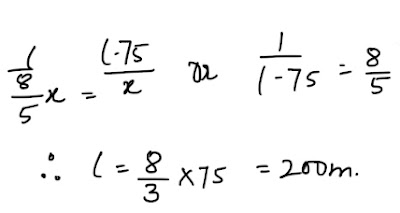# Races and Games of Skills : Important Questions for the Exam#### Ques 1.

A is 1 3/5 times quicker than B. A gives B a start of 75 metres, find the length of racecourse so that both of them reach the winning post at the same time.
Sol. Let the length of Racecourse be/m.
. . In a time in which A covers i m, B covers (I-75)m
let speed of B be x. Speed A = 8/5 x As time is same for both we have :#### Ques 2.

In a game of snooker A can give B 16 points in 80 and A can give C 15 points in 90.How many points can C give B in a game of 50 ?
Sol.  When A 80 points B scores 64 points.
When A scores 90 points B scores 64/80x90 points = 72 points.
i.e. when C scores 75 points B scores 72 points.
.. When C scores 50 points B scores 72/75 x 50 points = 48 points .
Hence c give B 2 points in a game of 50.

#### Ques 3.

P can run 100 m in 20 seconds and Q in 25 seconds .P beats Q by.
(a) 10 m
(b) 20 m
(c) 25 m
(d) 12 m
Sol.  20 m
Hint: In 20 seconds Q runs = 100/25 x 20 m

#### Ques 4.

In a 2 km race, P can give Q 200 m and R 560 m. In the same race, Q can give R
(a) 400 m
(b) 300 m
(c) 350 m
(d) 500 m
Sol.  400 m
Hint : When Q runs ( 2000-200)m, R suns (2000-560)m.

#### Ques 5.

A runs 1.5 times as fast as B can. If A gives B a start of 50m, how far must the winning post be in order that A and B reach at the same time ?
(a) 150 m
(b) 120 m
(c) 125 m
(d) 180 m
Sol.  150 m
Hint : d-50/s   = d/1.5s

#### Ques 6.

P and Q run a kilometre and P wins by 1 minute . P and R run a kilometre and P wins by 375 m. Q and R run a kilometre and Q wins by 30 seconds. Find the time taken by each runner to run a kilometre.
(a) 150 sec, 210 sec, 240 sec
(b) 120 sec, 160 sec, 200 sec
(c) 100 sec, 160 sec, 190 sec
(d) 140 sec, 200 sec, 230 sec
Sol. 150 sec, 210 sec, 240 sec
Hint : Let teh speed of  P ,Q  and R be p,q and r ms-1 respectively.#### Ques 7.

A can give B 25 points,A can give C 40 points and B can give C 20 points .How many points make of the game ?
(a) 120
(b) 100
(c) 150
(d) 80
Sol.  100
Hint : Let the game be of X points.
when A scores x points, B scores x -25 points, and C  scores X - 40 points.

#### Ques 8.

At a game of billiards, A can give B 12 points in a game of 40 and a can give C 10 points in a game of 50. How many points can C give B in a game of 80 ?
(a) 10
(b) 20
(c) 12
(d) 18
Sol. 10
Hint: When a scores 40 points, B scores ( 40-12) points.
When A scores 50 points , C scores ( 50-10) points.#### What's trending in BankExamsToday

Smart Prep Kit for Banking Exams by Ramandeep Singh - Download here